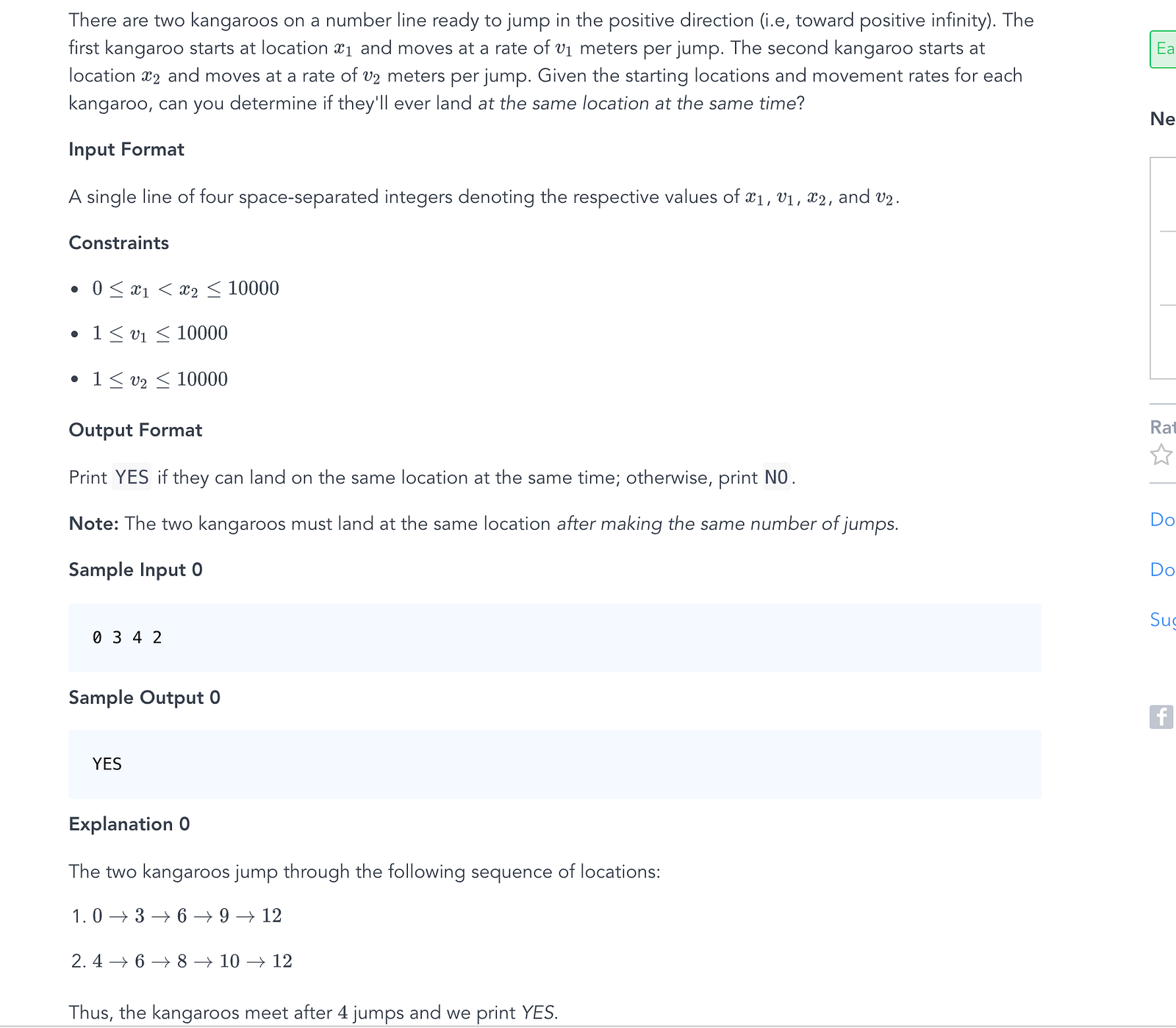# How important is math in computer programming?

8 January 2018I’m writing this article because I recently did this exercise in HackerRank:I don’t have a degree in computer science or similar (but i’m working as a software engineer the last 3 years) so I really don’t have a solid math knowledge and at first sight this exercise seems easy, right?

Most of you will think that it is, but to me it wasn’t. My first approach was to think in a logical solution (at least I’d like to think that it was), then I thinked that if i wanted to know if the kangaroos will ever land on the same location at the same time I would have to move the kangaroos until they were both on the same location

I designed a loop that would move the kangaroos and check if they are on the same position.

Forgetting other validations, I only wrote the necessary code for the case:

``````for i:=0 ; i<10000; i++ {
kOnePosition = moveKangoro(x1, v1)
kTwoPosition = moveKangoro(x2, v2)
if kOnePosition == kTwoPosition {
fmt.Println(“YES”)
break;
}
}
``````

I wrote variants of this code and other ones, but neither of them passed the tests. After 10 or 20 minutes I thought in a math solution.

The equation to verify if in n jumps the kangaroos are going to be on the same location is this:

x1 + (v1 * n) = x2 + (v2 * n) -> where n is of num of jumps.

So, we are going to resolve this equation:

(v1 * n) — (v2 * n) = x2 — x1

n(v1 — v2) = x2 — x1

n = (x2 — x1) / (v1 — v2)

We know that the value of n needs to be an integer, so we’re going to replace the division by the modular division and check if the operation leaves a remainder of 0

(x2 — x1) % (v1 — v2) == 0

This means that for a set of initial positions and meters per jump, we can know that the kangaroos are going to be on the same position at the same time only if the remainder value is 0.

Again, I’m forgetting other validations, I only wrote the necessary code for the case:

``````if x1 == x2 && v1 == v2 {
fmt.Println("YES")
} else if x1 == x2 && v1 != v2{
fmt.Println("NO")
} else {
if v1 > v2 && ((x2-x1)%(v1-v2)) == 0 {
fmt.Println("YES")
} else {
fmt.Println("NO")
}
}
``````

This algorithm passed all the tests and we could only do this efficiently with maths.

Sometimes I hear developers say “Math is not very important” or phrases like “A good coder is bad at math” and I think that they are wrong because as we can saw in this case, maths helps us to solve a problem in an efficient way.

I’ve a lot to learn and It’s exciting to find these problems to improve every day.

Code in Github

Share article